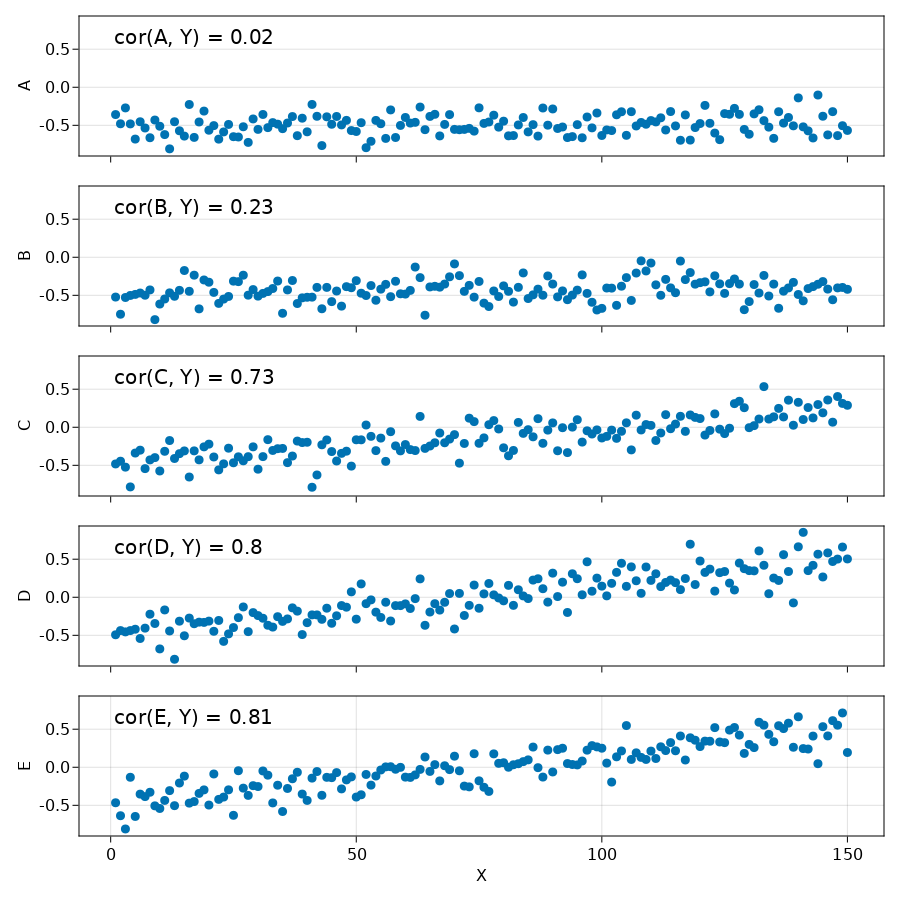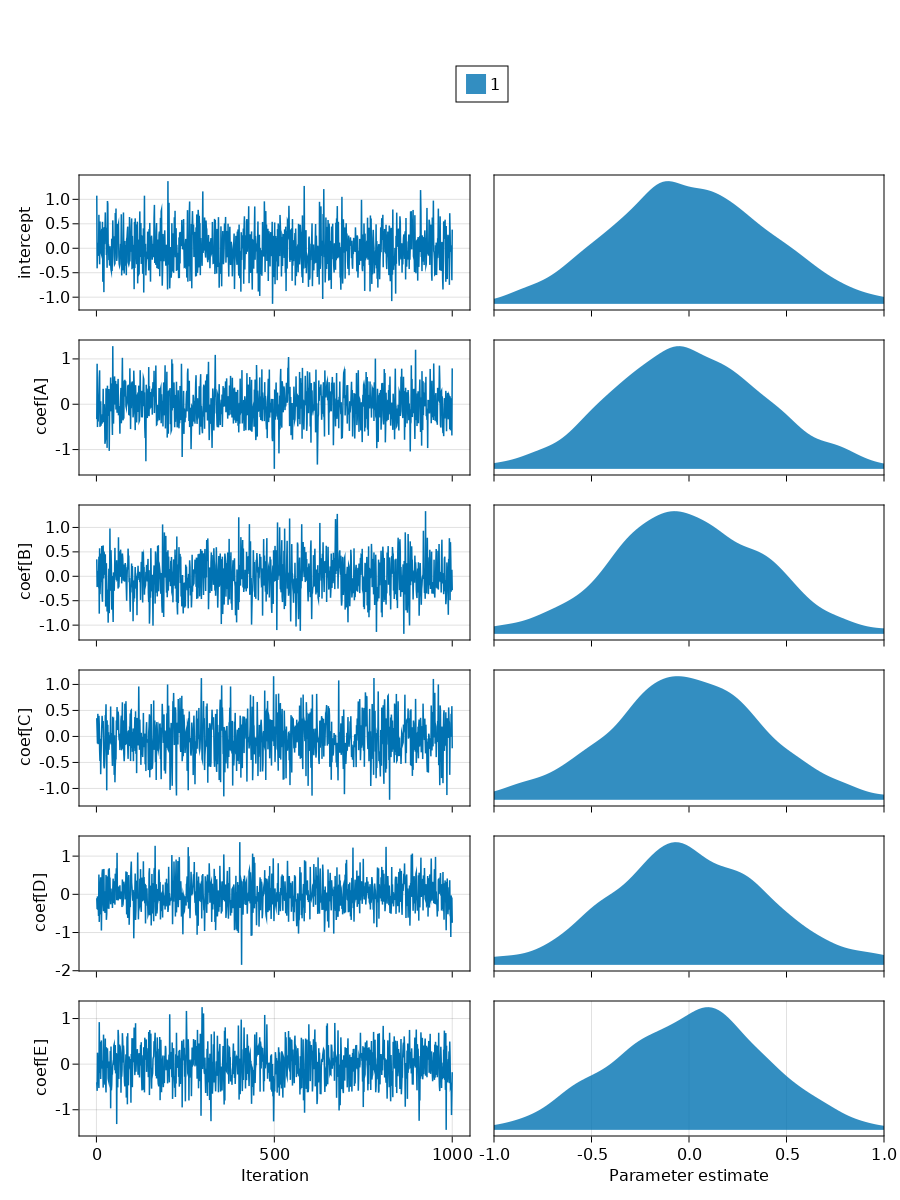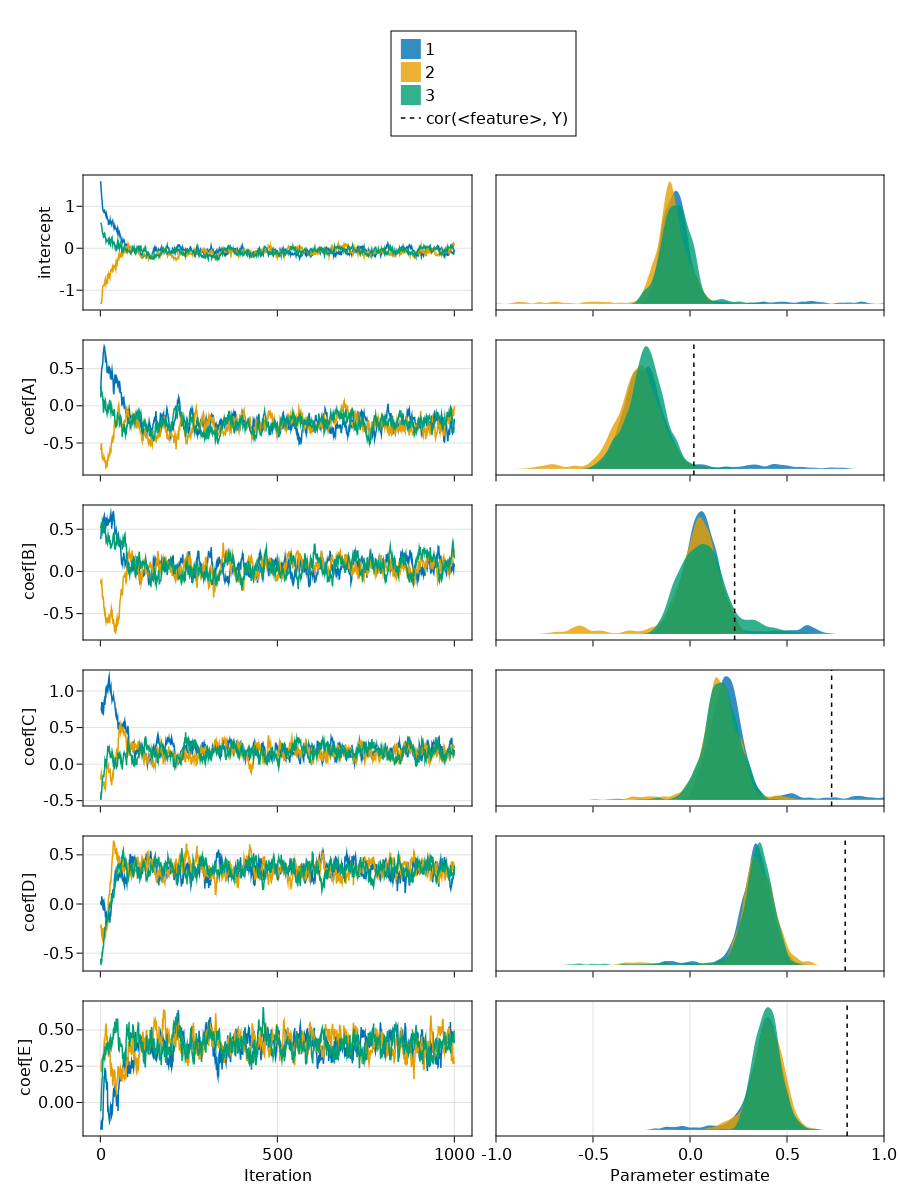In my post on Shapley values and multicollinearity, I looked into what happens when you fit a complex uninterpretable model on collinear or near-collinear data and try to figure out which features (variables) are important. The results were reasonable but not great. Luckily, there are still more things to try. Gelman et al. (2020) say that Bayesian models can do reasonably well on collinear data because they show high uncertainty in the estimated coefficients. Also, Bayesian models have a chance of fitting the data better as is beautifully shown in the Stan documentation. It can be quite tricky to implement though because a good parameterization is necessary (https://statmodeling.stat.columbia.edu/2019/07/07/collinearity-in-bayesian-models/).

## Simulating data

Let's simulate some data with various columns are increasingly correlated with the outcome (and thus each other). Here, we assume that the data is centered around zero. This is easier for the Bayesian model to work with, but can often also make interpretation of the coefficients easier. There are various methods to rescale data, one is using `MLDataUtils: rescale!`. Note that `rescale!` bases the rescaling on the sample which is not recommended for small samples (Gelman, 2020). Instead, you can use knowledge that you have about the data such as the range of questionnaire scores or the weight of cars. Specifically, for example, it could be known for the data that the weight of a car is never below zero and unlikely to be above 3600 kg (8000 lbs); the weight of a Hummer H1.

``````begin
using CairoMakie
using CategoricalArrays: categorical
using DataFrames: Not, DataFrame, select, stack, transform
using GLM
using Turing
using Random: seed!
using Statistics: rand, mean, cor
end``````
``indexes = 1.0:150.0;``
``y_true(x) = x / last(indexes);``
``y_noise(x, corr_coefficient) = (corr_coefficient * y_true(x) - 0.5) + rand(Normal(0, 0.15));``
``````df = let
seed!(0)
X = indexes
A = y_noise.(indexes, 0)
B = y_noise.(indexes, 0.05)
C = y_noise.(indexes, 0.7)
D = y_noise.(indexes, 1)
E = y_noise.(indexes, 1)
Y = y_noise.(indexes, 1)

DataFrame(; X, A, B, C, D, E, Y)
end``````
X A B C D E Y
1.0 -0.358554 -0.522957 -0.481998 -0.492793 -0.466424 -0.552454
2.0 -0.479912 -0.748431 -0.445867 -0.437615 -0.636698 -0.931691
3.0 -0.27124 -0.5285 -0.524026 -0.45503 -0.810672 -0.369753
4.0 -0.481415 -0.50173 -0.783662 -0.437662 -0.131537 -0.0454284
5.0 -0.680866 -0.488108 -0.338793 -0.420014 -0.646305 -0.390959
6.0 -0.453227 -0.469888 -0.300942 -0.541693 -0.352914 -0.672081
7.0 -0.535196 -0.498834 -0.543361 -0.405727 -0.385467 -0.324522
8.0 -0.663103 -0.429192 -0.428918 -0.22254 -0.328334 -0.524071
9.0 -0.430653 -0.819506 -0.398711 -0.344799 -0.506967 -0.501721
10.0 -0.512089 -0.614823 -0.574396 -0.678601 -0.541517 -0.357891
...
150.0 -0.567046 -0.421028 0.288692 0.50339 0.19394 0.328947

The data and the correlations look as follows:## Defining the model

This is a basic linear regression model similar to the one mentioned in the tutorials on https://turing.ml. The priors of this model are visualized below.

``````@model function linear_regression(X::Matrix, y)
σ₂ ~ truncated(Normal(0, 100), 0, Inf)

intercept ~ Normal(0, 0.4)

n_features = size(X, 2)
coef ~ MvNormal(n_features, 0.4)

mu = intercept .+ X * coef
y ~ MvNormal(mu, sqrt(σ₂))
end;``````
``X = select(df, Not([:X, :Y]));	``
``````model = let
y = df.Y
model = linear_regression(Matrix(X), y)
end;``````

# Inspecting the prior

To verify that the priors are correctly set, we can use `sample(model, Prior(), n_samples)` from Turing.jl. This is shown below with the raw sample values on the left and the density plot for these values on the right.

``n_samples = 1_000;``In this plot, everything looks good. On average, we expect our data to be zero (centered) and the variance looks reasonable. We expect the coefficients for the linear model to be between -0.5 and 0.5. Thanks to these priors, the sampler should have useful samples right from the start.

``````function mysample(model, sampler)
n_chains = 3
chns = sample(model, sampler, MCMCThreads(), n_samples, n_chains)
return fix_names(chns)
end;``````

## Estimating the parameters

When we fit the model, we have to decide on a sampler for this complex collinear case. NUTS is normally the best bet in Turing.jl, but let's first try HMC.

In the plots below, the different colors indicate different chains. All plots show good mixing and stationarity on the leftmost plots; the chains properly converged to the same outcome:

``````let
chns = mysample(model, HMC(0.005, 10))
plot_chain(chns)
end``````Obtaining this outcome required setting the leapfrog size to a very low number. Normally, it is 0.05 or 0.1 which both did not work. What I mean by did not work is that the different chains did not converge, that is, gave different outcomes. Note that, thanks to the low leapfrog size, it took quite a few iterations for the chains to converge.

Let's try a more modern sampler, namely NUTS:

``````let
chns = mysample(model, NUTS())
plot_chain(chns)
end``````